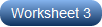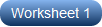# Nature of Roots Worksheets

Is it possible to determine if the roots of a quadratic equation are real or complex without solving it? It's definitely a yes. Not only that you can do this, but you can also predict if the roots are equal or unequal. Here's how: you can tell about the nature of the roots by evaluating the discriminant (delta), Δ = b2 - 4ac upon plugging in it, a, b, and c of the quadratic equation ax2 + bx + c = 0. If Δ = 0, the roots are real and equal; if Δ > 0, the roots are real and unequal; if Δ < 0, the roots are unreal or complex. Now, there's another question for you. What if the nature of roots is given, and you are asked to find the values or at least the range of a, b, and c in the equation? Can you answer? Acquire and augment both these skills with our printable nature of roots worksheets, promising two broad levels of practice pdfs for high school students. Begin your practice with our free worksheet!

Find the Nature of Roots - Easy

Kick into gear with this set of easy exercises presenting ten simple quadratic equations for practice. Isolate the integer coefficients and constant from the equation, substitute them and calculate Δ, and determine the nature of roots.Find the Nature of Roots - Moderate

Usher in a new era of confidence among high school students with these pdf worksheets. Handing out a moderate practice, the quadratic equations in here have fractional and radical coefficients apart from integers. Figure out Δ and determine the nature of roots.Find the Values of Unknown

Just to get you work the other way around, the nature of the roots of the quadratic equations are given and you need to find the possible or in some cases, exact values of the indicated unknowns. Set up the equation or inequality using Δ, and solve for the missing values.